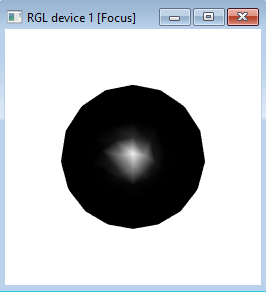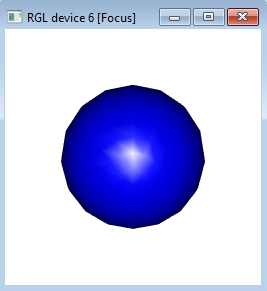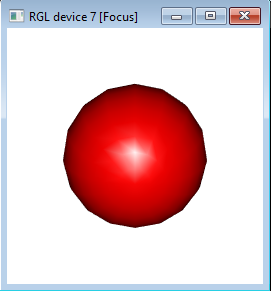# How to create a sphere in R?

To create a sphere, we can use spheres3d function of rgl package. The rgl package is specifically designed to create real-time interactive 3D plots. If we want to create a sphere then we need to pass the values for the three axes and the radius of the sphere. We can also change the color of the sphere by introducing color argument inside spheres3d function.

## Example1

> library(rgl)
> spheres3d(x=1,y=1,z=1,radius=1)

## Output:## Example2

> spheres3d(0,0,0,radius=1,color="blue")

## Output:## Example3

> spheres3d(0,0,0,radius=1,color="red")

## Output: